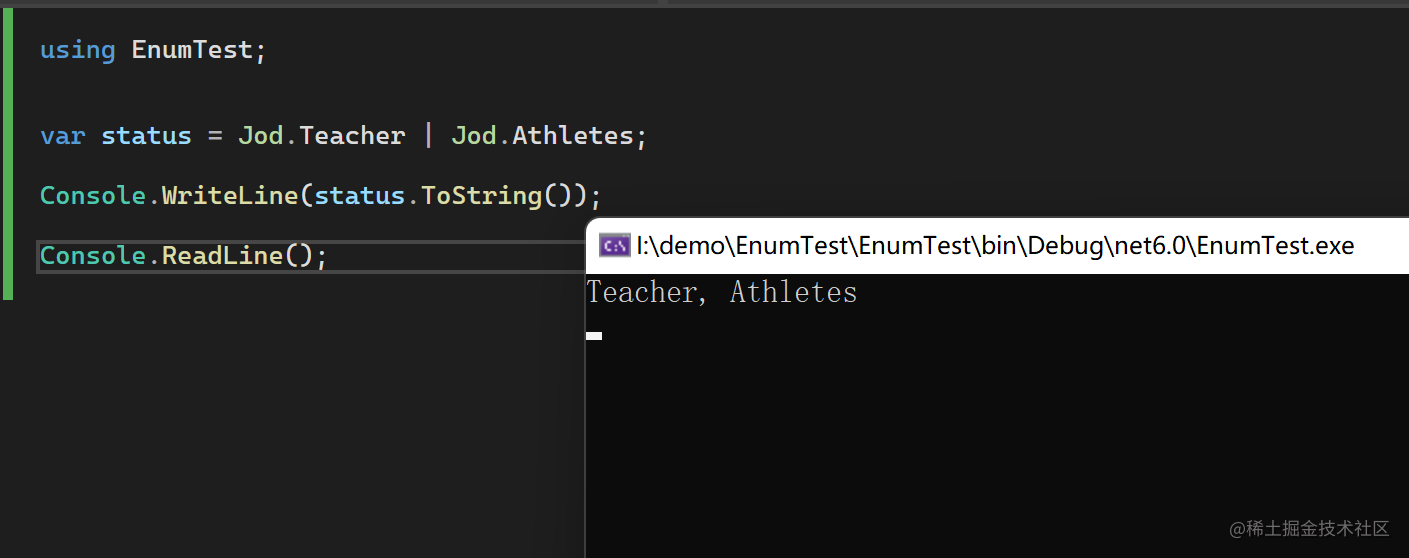2022/04/25 11:55

# 基本使用

public enum OrderStatus
{
/// <summary>
/// 未支付
/// </summary>
WaitPay = 0,

/// <summary>
/// 已支付
/// </summary>
Payed = 1,

/// <summary>
/// 已退款
/// </summary>
Refund = 2,

/// <summary>
/// 已关闭
/// </summary>
Closed = 3,
}


public enum OrderStatus: byte
{
/// <summary>
/// 未支付
/// </summary>
WaitPay = 0,

/// <summary>
/// 已支付
/// </summary>
Payed = 1,

/// <summary>
/// 已退款
/// </summary>
Refund = 2,

/// <summary>
/// 已关闭
/// </summary>
Closed = 3,
}


# 搭配Description使用

public enum OrderStatus
{
[Description("未支付")]
WaitPay = 0,

[Description("已支付")]
Payed = 1,

[Description("已退款")]
Refund = 2,

[Description("已关闭")]
Closed = 3,
}


public static class EnumExtensions
{
public static string GetDescription(this Enum obj)
{
object[]? array = obj.GetType().GetField(obj.ToString())?.GetCustomAttributes(typeof(DescriptionAttribute), inherit: true);
if (array != null)
{
var attr = array.FirstOrDefault(x => x is DescriptionAttribute);
if (attr != null)
{
return ((DescriptionAttribute)attr).Description;
}

}

return string.Empty;
}
}


# 搭配Flag属性使用

internal enum Jod
{
/// <summary>
/// 老师
/// </summary>
Teacher = 1,

/// <summary>
/// 运动员
/// </summary>
Athletes = 2
}


[Flags]
internal enum Jod
{
/// <summary>
/// 老师
/// </summary>
Teacher = 1,

/// <summary>
/// 运动员
/// </summary>
Athletes = 2
}# 位运算

[Flags]
internal enum Jod
{
/// <summary>
/// 老师
/// </summary>
Teacher = 1,

/// <summary>
/// 运动员
/// </summary>
Athletes = 2,

/// <summary>
/// 歌手
/// </summary>
Singer = 3,

/// <summary>
/// 舞者
/// </summary>
Dancer = 4
}


[Flags]
internal enum Jod
{
/// <summary>
/// 老师
/// </summary>
Teacher = 1,

/// <summary>
/// 运动员
/// </summary>
Athletes = 2,

/// <summary>
/// 歌手
/// </summary>
Singer = 4,

/// <summary>
/// 舞者
/// </summary>
Dancer = 8,

Jobx = 0x10,

JobY = 0x20,

JobZ = 0x40,
...
}


// 1.基本的或运算，表示同时有多种枚举值的情况
var jobs = Jod.Teacher | Jod.Athletes;

// 2.判断某个人的职业中是否有Athletes
if ((jobs & Jod.Athletes) == Jod.Athletes)
{
// 是运动员
}



# 数据库设计中的妙用

## 一般场景

merch_id 商户id long
merch_name 商户名 string
certified 已认证? int(0或1)

## 优化

merch_id 商户id long
merch_name 商户名 string
merch_flags 各种商户标识 int

[Flags]
public enum MerchFlags
{
/// <summary>
/// 已认证?
/// </summary>
certified = 1,
/// <summary>
/// vip商户?
/// </summary>
is_vip_merc = 2,
/// <summary>
/// 商品上架免检
/// </summary>
is_defect_free = 4,
/// <summary>
/// 是否冻结
/// </summary>
s_frozen = 8,
/// <summary>
/// 是否金牌商户
/// </summary>
is_mvp = 0x10,

// ...继续新增各种标志位
}


## 思考一个问题### 作者的其它热门文章

0
0 收藏

0 评论
0 收藏
0